# A charge +q is at the origin. A charge -2q is at x = 1.30 m on the +x axis.(a) For what finite value of x is the electric field zero?(b) For what finite values of x is the electric potential zero? (Note: Assume a reference level of potential V = 0 at r = 0,.)Smallest value of x:-1.3Largest value of x:

Question
17 views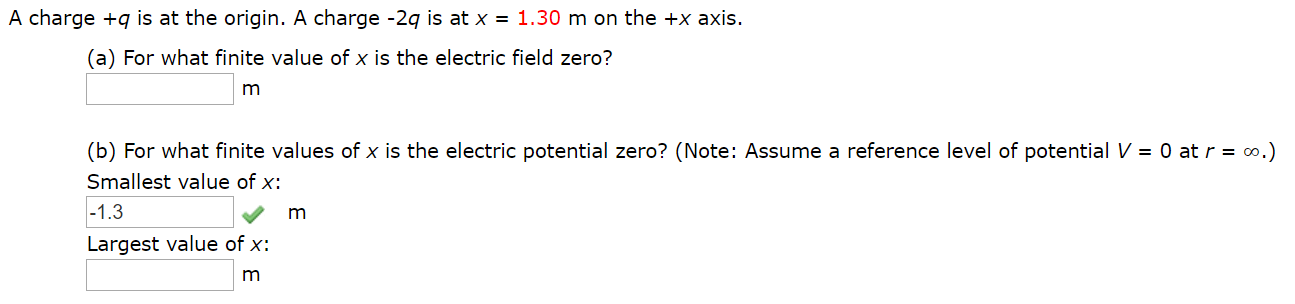help_outlineImage TranscriptioncloseA charge +q is at the origin. A charge -2q is at x = 1.30 m on the +x axis. (a) For what finite value of x is the electric field zero? (b) For what finite values of x is the electric potential zero? (Note: Assume a reference level of potential V = 0 at r = 0,.) Smallest value of x: -1.3 Largest value of x: fullscreen
check_circle

Step 1

(a)

The figure shows the charge is located on the +x axis.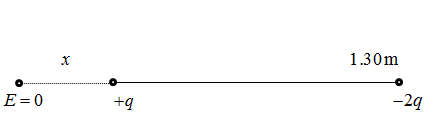From the figure, the electric field where the point zero at a finite distance,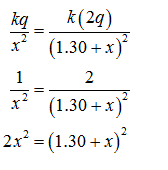Step 2

Solve the above relation for quadratic equation.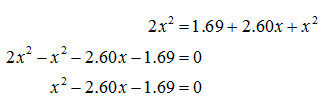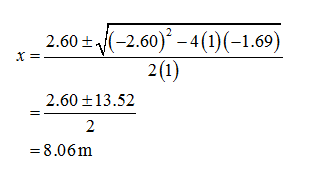Hence, finite value of x at which the electric fields zero taken as negative value is -8.06 m.

Step 3

(b)

The figure shows the charge is located on the +x axis.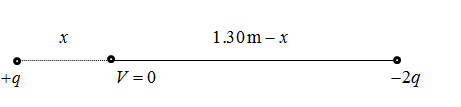From the figure, the electric potential where the point zero at a finite distance,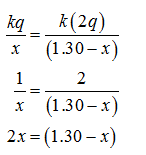...

### Want to see the full answer?

See Solution

#### Want to see this answer and more?

Solutions are written by subject experts who are available 24/7. Questions are typically answered within 1 hour.*

See Solution
*Response times may vary by subject and question.
Tagged in

### Physics